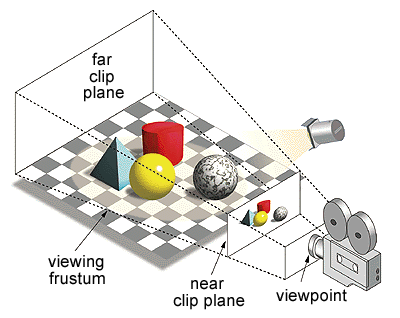# Frustum

The frustum structure is actually an acceleration structure. It's a primitive shape (Like an AABB or any other primitive). A frustum structure is usually built out of a view matrix as a series of 6 planes.## The Algorithm

If this all sounds a bit familiar, it should. We have actually done this exact same thing when we made a frustum in OpenGL.

To extract the planes of a frustum, you need the viewProjection matrix, which you get by multiplying the projection and view matrices together. The plane values can be found by adding or subtracting one of the first three rows of the viewProjection matrix from the fourth row.

Our camera class already has the ViewMatrix, but not the projection matrix! Let's fix that. Add the following getter to your Camera class. This getter will simply query OpenGL for the current projection matrix. This is similar to what we did in the picking example.


public Matrix4 ProjectionMatrix {
get {
float[] rawProjection = new float;
GL.GetFloat(GetPName.ProjectionMatrix, rawProjection);
return Matrix4.Transpose(new Matrix4(rawProjection));
}
}


Now that we have the projection matrix, we can resume extracting the planes

• Left Plane Row 1 (addition)
• Right Plane Row 1 Negated (subtraction)
• Bottom Plane Row 2 (addition)
• Top Plane Row 2 Negated (subtraction)
• Near Plane Row 3 (addition)
• Far Plane Row 3 Negated (subtraction)

So, assuming that matrix mp is the view-projection matrix, you could get the left and right planes like so:

Matrix4 mv =  ProjectionMatrix * ViewMatrix;

Vector4 row1 = new Vector4(mv[0, 0], mv[0, 1], mv[0, 2], mv[0, 3]);
Vector4 row4 = new Vector4(mv[3, 0], mv[3, 1], mv[3, 2], mv[3, 3]);

Vector4 p1 = row4 + row1;
Vector4 p2 = row4 - row1;

Plane left = new Plane();
left.n = new Vector3();
left.n.X = p1.X;
left.n.Y = p1.Y;
left.n.Z = p1.Z;
left.d = p1.w;

Plane right = new Plane();
right.n = new Vector3();
right.n.X = p2.X;
right.n.Y = p2.Y;
right.n.Z = p2.Z;
right.d = p2.w;


## Fixing some things

Originally, the Frustum getter was a On Your Own todo. However, i noticed a pretty big error with the way we did it previously on our OpenGL Implementation. So, i'm just going to provide the code for it and explain what was wrong. Add the following code to the Camera class:

private static Plane FromNumbers(Vector4 numbers) {
Vector3 abc = new Vector3(numbers.X, numbers.Y, numbers.Z);
float mag = abc.Length();
abc.Normalize();

Plane p = new Plane();
p.Normal = abc;
p.Distance = numbers.W / mag;
return p;
}

public Plane[] Frustum {
get {
Plane[] frustum = new Plane;

Matrix4 vp = ProjectionMatrix * ViewMatrix;

Vector4 row1 = new Vector4(vp[0, 0], vp[0, 1], vp[0, 2], vp[0, 3]);
Vector4 row2 = new Vector4(vp[1, 0], vp[1, 1], vp[1, 2], vp[1, 3]);
Vector4 row3 = new Vector4(vp[2, 0], vp[2, 1], vp[2, 2], vp[2, 3]);
Vector4 row4 = new Vector4(vp[3, 0], vp[3, 1], vp[3, 2], vp[3, 3]);

frustum = FromNumbers(row4 + row1);
frustum = FromNumbers(row4 - row1);
frustum = FromNumbers(row4 + row2);
frustum = FromNumbers(row4 - row2);
frustum = FromNumbers(row4 + row3);
frustum = FromNumbers(row4 - row3);

return frustum;
}
}


PReviously, the FromNumbers function was busted. It was not setting the plane normal to a normalized number. Also, when normalizing, you MUST divide the W component by the length of the XYZ components. PReviously, that was not being done either. The above version is correct! In order for the below unit test to pass, you have to populate your frustum as follows:

• frustum = left plane
• frustum = right plane
• frustum = bottom plane
• frustum = top plane
• frustum = near plane
• frustum = far plane

### Unit Test

Add the following unit test at the bottom of the Initialize function of CameraSample

Plane[] frustum = new Plane;
frustum = new Plane(new Vector3(0.2381448f, -0.2721655f, -1.598973f), 44.82524f);
frustum = new Plane(new Vector3(-1.598973f, -0.2721655f, 0.2381448f), 44.82524f);
frustum = new Plane(new Vector3(-1.013747f, 1.394501f, -1.013747f), 36.74234f);
frustum = new Plane(new Vector3(-0.3470805f, -1.938832f, -0.3470805f), 36.74234f);
frustum = new Plane(new Vector3(-1.360841f, -0.5443366f, -1.360841f), 73.4747f);
frustum = new Plane(new Vector3((float)1.364946E-05, (float)5.453825E-06, (float)1.364946E-05), 923.8687f);

for (int i = 0; i < 6; ++i) {
if (camera.Frustum[i].Normal != frustum[i].Normal) {
if (Vector3.Normalize(camera.Frustum[i].Normal) == Vector3.Normalize(frustum[i].Normal)) {
System.Console.WriteLine("\tLooks like the normal of your frustum plane is NOT NORMALIZED!");
}
else {
System.Console.WriteLine("Detected error in frustum, plane " + i);
}
System.Console.WriteLine("\tExpected: " + camera.Frustum[i].Normal);
System.Console.WriteLine("\tGot: " + frustum[i].Normal);
}
if (System.Math.Abs(camera.Frustum[i].Distance - frustum[i].Distance) > 0.0001f) {
System.Console.WriteLine("Wrong distance for plane: " + i);
System.Console.WriteLine("\tExpected: " + camera.Frustum[i].Distance);
System.Console.WriteLine("\tGot: " + frustum[i].Distance);
}
}


All tests should pass before moving on to the next section.